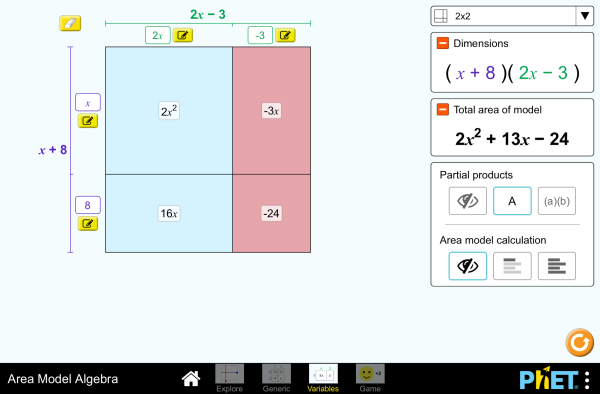# 面積と式の展開ダウンロード組み込む 閉じる このシミュレーションの実行可能なコピーを組み込む このシミュレーションの実行可能なコピーを組み込むためには、このHTMLコードをお使いください。HTMLコード内の"width"と"height"の属性を変更することによって、組み込まれたシミュレーションの幅と高さを調整できます。 シミュレーション起動用の画像を組み込む
クリックして実行
「クリックして実行」と書かれたスクリーンショットを表示するためには、このHTMLコードをお使いください。 多項式 Factors 生成物 PhETの支援者あなたの様な教育者の方々

• 多項式
• Factors
• 生成物

### Description

Build rectangles of various sizes and relate multiplication to area. Discover new strategies for multiplying algebraic expressions. Use the game screen to test your multiplication and factoring skills!

### 学習目標例

• Develop and justify a method to use the area model to determine the product of a monomial and a binomial or the product of two binomials.
• Factor an expression, including expressions containing a variable.
• Recognize that area represents the product of two numbers and is additive.
• Represent a multiplication problem as the area of a rectangle, proportionally or using generic area.
• Develop and justify a strategy to determine the product of two multi-digit numbers by representing the product as an area or the sum of areas.

### Standards Alignment

#### Common Core - Math

3.MD.C.7
Relate area to the operations of multiplication and addition.
3.MD.C.7c
Use tiling to show in a concrete case that the area of a rectangle with whole-number side lengths a and b + c is the sum of a × b and a × c. Use area models to represent the distributive property in mathematical reasoning.
3.MD.C.7d
Recognize area as additive. Find areas of rectilinear figures by decomposing them into non-overlapping rectangles and adding the areas of the non-overlapping parts, applying this technique to solve real world problems.
4.NBT.B.5
Multiply a whole number of up to four digits by a one-digit whole number, and multiply two two-digit numbers, using strategies based on place value and the properties of operations. Illustrate and explain the calculation by using equations, rectangular arrays, and/or area models.
6.EE.A.3
Apply the properties of operations to generate equivalent expressions. For example, apply the distributive property to the expression 3 (2 + x) to produce the equivalent expression 6 + 3x; apply the distributive property to the expression 24x + 18y to produce the equivalent expression 6 (4x + 3y); apply properties of operations to y + y + y to produce the equivalent expression 3y.
6.EE.A.4
Identify when two expressions are equivalent (i.e., when the two expressions name the same number regardless of which value is substituted into them). For example, the expressions y + y + y and 3y are equivalent because they name the same number regardless of which number y stands for..
6.NS.B.4
Find the greatest common factor of two whole numbers less than or equal to 100 and the least common multiple of two whole numbers less than or equal to 12. Use the distributive property to express a sum of two whole numbers 1-100 with a common factor as a multiple of a sum of two whole numbers with no common factor. For example, express 36 + 8 as 4 (9 + 2)..
7.EE.A.1
Apply properties of operations as strategies to add, subtract, factor, and expand linear expressions with rational coefficients.
7.NS.A.2c
Apply properties of operations as strategies to multiply and divide rational numbers.
8.EE.C.7b
Solve linear equations with rational number coefficients, including equations whose solutions require expanding expressions using the distributive property and collecting like terms.
Version 1.2.1

### Teacher Tipsインストラクター用ガイド（pdf）には、PhETチームが作成したヒント集が含まれています。 ( PDF ).

### Teacher-Submitted Activities

Games Remote Lesson ideasTrish Loeblein 大学基礎

Remote

Expanding and Factoring Algebraic ExpressionsAmanda McGarry 中学 Guided 数学
Algebraic ExpressionsAmanda McGarry 中学 Guided 数学
Exploring Factoring as Undoing the Distributive PropertySarah Hampton 中学

Guided 数学
SECUNDARIA: Alineación PhET con programas de la SEP México (2011 y 2017)Diana López 高校

その他 数学

PREPARATORIA: Alineación de PhET con programas de la DGB México (2017)Diana López 大学基礎

その他 物理学

Middle School Math Sim AlignmentAmanda McGarry 中学 その他 数学
Using the area model with expressionsJennifer Knudsen, Teresa Lara-Meloy, Amanda McGarry 中学 Discuss 数学
Modelo de Áreas, Cambio de Expresiones Andrés Gualberto Palomo Cuevas 高校 宿題
Remote

Would You Rather ... Karen Gann 高校 Guided 数学
Area Model Algebra - Multiplying Polynomials Carolyn Felknor 中学 Guided 数学
ARTICULANDO ÁLGEBRA E GEOMETRIA: O CASO DAS EQUAÇÕES ALGÉBRICAS NO ESTUDO DE ÁREAS Verusca Batista Alves; Ana Carolina Costa Pereira 中学 宿題

Distributive Property Review Tim Kissinger and Larry Peters 中学 Guided
Discuss

アラビア語 All العربيةجبر نموذج المساحة
アルバニア語 All shqipModeli Syprinës: Algjebër
イタリア語 All italianoRappresentazione grafica della moltiplicazione in algebra
ウクライナ語 All українськаПлощинна модель. Алгебраオランダ語 All NederlandsAlgebra van oppervlakte
カザフ語 All KazakhАуданды есептеу
ギリシャ語 All ΕλληνικάΥπολογισμός εμβαδού
グジャラート語 All Gujaratiક્ષેત્રફળ મોડેલ - બીજગણિત
シンハラ語 All Sinhaleseවර්ගඵල ආකෘති වීජ ගණිතය
スウェーデン語 All svenskaAreamodellen och algebra
スペイン語 All españolModelo de Áreas: Álgebraスペイン語（ペルー） All español (Perú)Modelo de Área con Álgebra
スペイン語（メキシコ） All español (México)Modelo de Áreas: Álgebraセルビア語 All СрпскиМатематика преко површина - Алгебра
タミル語 All Tamilமாதிரி உருவின் எண்(அட்சர) கணிதம்
デンマーク語 All DanskArealmodel, algebra
ドイツ語 All DeutschFlächenmodell Algebra
トルコ語 All TürkçeAlan Modeli İle Cebir
バスク語 All EuskaraAzalera-modeloan algebra
ハンガリー語 All magyarTerületmodell: algebra
ヒンディー語 All हिंदीबीजगणित -नमूने का क्षेत्रफल
フィンランド語 All suomiPinta-ala ja algebra
ブラジルポルトガル語 All português (Brasil)Modelo de Área: Álgebra
フランス語 All françaisModélisation par les aires - Algèbre
ベトナム語 All Tiếng ViệtĐại số với mô hình diện tích
ペルシャ語 All فارسیجبر مدل مساحت
ボスニア語 All BosanskiMatematički model površina - Algebra
ポルトガル語 All portuguêsModelo de Área: Álgebra
ポーランド語 All polskiModel pola - wyrażenia algebraiczne
マラッタ語 All मराठीक्षेत्रफळाचे बीजगणित
モンゴル語 All Монгол (Монгол)Талбайн загварын Алгебр
ラトビア語 All LatviešuLaukuma modeļa algebra
ロシア語 All русскийВычисление площади

HTML5 sims can run on iPads and Chromebooks, as well as PC, Mac, and Linux systems.

iOS 12+ Safari

Android:
Not officially supported. If you are using the HTML5 sims on Android, we recommend using the latest version of Google Chrome.

Chromebook:
The HTML5 and Flash PhET sims are supported on all Chromebooks.
Chromebook compatible sims

Windows Systems:

Macintosh Systems:

Linux Systems:

デザインチーム サードパーティのライブラリ 謝辞
• Jonathan Olson (developer)
• Karina Hensberry
• Susan Miller
• Ariel Paul
• Kathy Perkins
• Mariah Hermsmeyer (artwork)
• Diana López Tavares (artwork)
• almond-0.2.9.js
• base64-js-1.2.0.js
• FileSaver-b8054a2.js
• font-awesome-4.5.0
• game-up-camera-1.0.0.js
• he-1.1.1.js
• himalaya-0.2.7.js
• jama-1.0.2
• jquery-2.1.0.js
• lodash-4.17.4.js
• pegjs-0.7.0.js
• seedrandom-2.4.2.js
• text-2.0.12.js
• TextEncoderLite-3c9f6f0.js